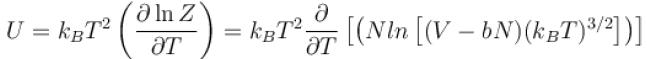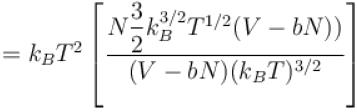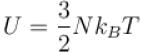Courses

# Thermodynamic Potential NAT Level – 2

## 10 Questions MCQ Test Topic wise Tests for IIT JAM Physics | Thermodynamic Potential NAT Level – 2

Description
This mock test of Thermodynamic Potential NAT Level – 2 for Physics helps you for every Physics entrance exam. This contains 10 Multiple Choice Questions for Physics Thermodynamic Potential NAT Level – 2 (mcq) to study with solutions a complete question bank. The solved questions answers in this Thermodynamic Potential NAT Level – 2 quiz give you a good mix of easy questions and tough questions. Physics students definitely take this Thermodynamic Potential NAT Level – 2 exercise for a better result in the exam. You can find other Thermodynamic Potential NAT Level – 2 extra questions, long questions & short questions for Physics on EduRev as well by searching above.
*Answer can only contain numeric values
QUESTION: 1

### For black body radiation, entropy varies with temperature as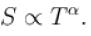Find the value of α?

Solution: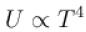(Stefan-Boltzman's lane)*Answer can only contain numeric values
QUESTION: 2

### For a fermi gas (in 8 dimension) value of the ratio Cp/CV is?

Solution:

Average energy of Fermious is given by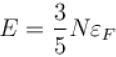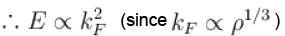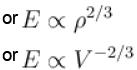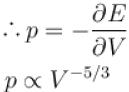pV5/3= constant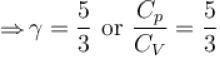*Answer can only contain numeric values
QUESTION: 3

### A quantity of heat ΔH is transferred from a large heat reservoir at temperature T to another large heat reservoir at temperature T/2. The heat reservoir have such large capacities that there is no observable change in true temperature. The ratio of the entropy change of the entire system to the entropy change in heat reservoir at temperature T/2 is?

Solution: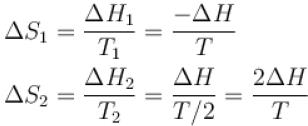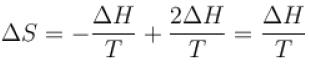Now,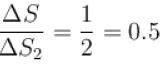*Answer can only contain numeric values
QUESTION: 4

At high temperature, entropy of a spin half system is equal to NkB time the natural logarithm of what number?

Solution:

zcosh(βμH) = 1 and μHβ[tanh(βμH)] = 0
∴ Entropy for spin 1/2 system which given by
S = Nk[ln{2cosh(βμH)} – βμHtanh(βμH)]
Reduces to S = NkBln 2

*Answer can only contain numeric values
QUESTION: 5

Virial of a system is defined as V = Σqipi , where qi is a generalized coordinate and pi conjugate momentum. Value of V for a system consisting of N particles at temperature T is equal to aNkBT. Find the value of constant a?

Solution:

Virial theorem states that
V = –2k·E
Since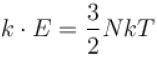⇒ V = –3NkT
⇒ a = –3

*Answer can only contain numeric values
QUESTION: 6

Entropy of a system of N classical harmonic oscillator having frequency ω and at temperature T is given by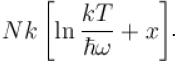Find the value of constant x?

Solution:

For Harmonic oscillator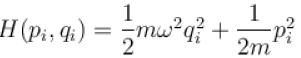∴ Single Oscillator partition function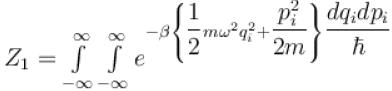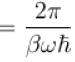∴ N-oscillator partition function
Z = (Z1 )N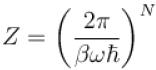∴ Helmholtz free energy function
F = –kTlnZ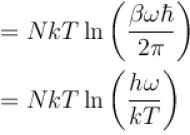∴ Entropy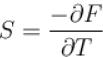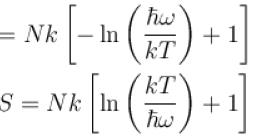∴ x = 1

*Answer can only contain numeric values
QUESTION: 7

The temperature of a cavity of fixed volume is doubled. Its energy increases by α times whereas number of photons increase by β times. Then calculate α/β?

Solution:

Energy of blackbody radiation αT4 and equilibrium number of photons in cavity αT3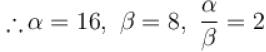*Answer can only contain numeric values
QUESTION: 8

For non interacting fermions entropy value with temperature for T → 0 as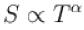. Find the value of α.

Solution:

For T → 0 internal energy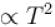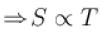⇒ α = 1

*Answer can only contain numeric values
QUESTION: 9

The mean internal energy of a one-dimensional harmonic oscillator in equilibrium with a heat bath of temperature T is in the units of kBT.

Solution: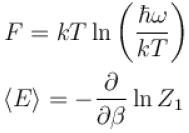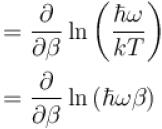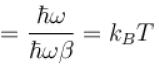*Answer can only contain numeric values
QUESTION: 10

A system of N particles is enclosed in a volume V at a temperature T. The logarithm of the partition function is given by ln Z = N ln{V – bN}(kBT)3/2 , where b is a constant with approximate dimensions. The internal energy of the gas in the units of NkBT is given as?

Solution: